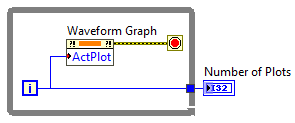LabVIEW

cancel
Showing results for
Did you mean:

How to get the number of plots in Waveform Graph or how to iterate plots of Waveform Graph?

I am using Labview 2017

I want to make a vi which get a waveform graph refnum and set the color of the plots

I understand how to change the color of plot using the Active plot and Plot color property when I know the index of the plot

But I don`t know how to iterate all plots of the Waveform Graph when I don`t know the number of plots

Is there any way to get the number of plots or iterate over the plots

Message 1 of 3
(192 Views)

Re: How to get the number of plots in Waveform Graph or how to iterate plots of Waveform Graph?

How did the plots get into the graph in the first place?

Somewhere in the program you created the graph.  In that part you put N sets of data into the graph.  Or put in a 2D array that you can get the dimensions of.  Determine that N and pass it to the part of the program where you are dealing with the colors either using a shift register, or a value property node or a local variable.

Message 2 of 3
(165 Views)

Re: How to get the number of plots in Waveform Graph or how to iterate plots of Waveform Graph?

This is the easiest way I know of to tell the number of plots in a waveform graph.DNatt, NI
Message 3 of 3
(160 Views)